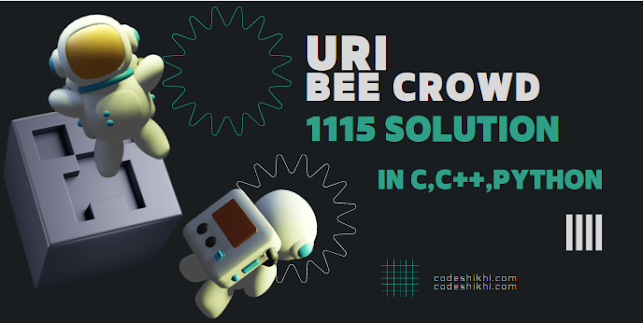# URI / BEE CROWD 1115 - Quadrant - Solution in C,C++,Python | URI - BEECROWD - BEE 1115 Solution in C,C++,Python# URI / BEE CROWD 1115 - Quadrant - Solution in C,C++,Python | URI - BEECROWD - BEE 1115 Solution in C,C++,Python

Write a program to read the coordinates (X, Y) of an indeterminate number of points in Cartesian system. For each point write the quadrant to which it belongs. The program finish when at least one of two coordinates is NULL (in this situation without writing any message).

## Input

The input contains several tests cases. Each test case contains two integer numbers.

## Output

For each test case, print the corresponding quadrant which these coordinates belong, as in the example.

 Input Sample Output Sample 2 23 -2-8 -1-7 10 2 primeiroquartoterceirosegundo

Demonstration:

This program takes user inputs. Then check the conditions for each test case contains.

Quadrant II: negative x and positive y

Quadrant III: negative x and negative y

Quadrant IV: positive x and negative y

N.B: Don't copy paste the code as same. Just try to understand it and try yourself.

## URI / BEECROWD Online Judge 1115 Solve in C :

```#include <stdio.h>
int main()
{
int a,b;
while(1)
{
scanf("%d%d", &a, &b);
if(a==0 || b==0)
break;
else if(a>0 && b>0)
printf("primeiro\n");
else if(a>0 && b<0)
printf("quarto\n");
else if(a<0 && b<0)
printf("terceiro\n");
else if(a<0 && b>0)
printf("segundo\n");
}
return 0;
}
```